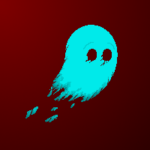I’ve put two codes one is for pastel colors only and is normal colors. You can apply this shader code to an colorect and change few parameters to your liking. If you want more in different or specific color types let me know in the comments. It is animated it moves from top to down.

Enjoy game dev 🙂

``````##Normal Colors

uniform float speed = 1.0;
uniform float frequency = 10.0;
uniform float amplitude = 0.1;

void fragment() {
vec2 uv = SCREEN_UV;
float time = TIME * speed;

float wave1 = sin(uv.x * frequency + time) * amplitude;
float wave2 = cos(uv.y * frequency + time) * amplitude;
uv += wave1 + wave2;

vec3 color1 = vec3(0.5 + 0.5 * sin(time), 0.5 + 0.5 * cos(time), 0.5 - 0.5 * sin(time));
vec3 color2 = vec3(0.5 - 0.5 * cos(time), 0.5 + 0.5 * sin(time), 0.5 + 0.5 * cos(time));
vec3 gradient_color = mix(color1, color2, uv.y);

}

##pastel colors

uniform float speed = 1.0;
uniform float frequency = 10.0;
uniform float amplitude = 0.1;

void fragment() {
vec2 uv = SCREEN_UV;
float time = TIME * speed;

// Create a water ripple effect
float wave1 = sin(uv.x * frequency + time) * amplitude;
float wave2 = cos(uv.y * frequency + time) * amplitude;
uv += wave1 + wave2;

// Generate constantly changing pastel colors using a gradient
vec3 color1 = vec3(0.8 + 0.2 * sin(time), 0.8 + 0.2 * cos(time), 0.8 - 0.2 * sin(time));
vec3 color2 = vec3(0.8 - 0.2 * cos(time), 0.8 + 0.2 * sin(time), 0.8 + 0.2 * cos(time));
vec3 gradient_color = mix(color1, color2, uv.y);

}``````
###### Tags
rippleThe shader code and all code snippets in this post are under CC0 license and can be used freely without the author's permission. Images and videos, and assets depicted in those, do not fall under this license. For more info, see our License terms.

## More from Miisannn

### Ripple effect

Subscribe
Notify of1 Comment
Inline Feedbacks